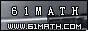Today is . WELCOME: huohai3983/44663  Home | Reg Login | All user | setHomePage | BookmarkHome | Math Test Online | Addition | Subtraction | Multiplication | Division | Time | Worksheet(JPG/PDF) | CAI | Flash Games |Recommendation articleArticle searchHome--maths--First Grade--Addition Facts for 3 and 4

Addition Facts for 3 and 4

When you add two numbers together you find how many you have in all.

The addition facts for three and four are listed in the following table.

 THREE 3+0=3 3+1=4 3+2=5 3+3=6 3+4=7 3+5=8 3+6=9 3+7=10 3+8=11 3+9=12 FOUR 4+0=4 4+1=5 4+2=6 4+3=7 4+4=8 4+5=9 4+6=10 4+7=11 4+8=12 4+9=13

5 + 3 = 8

 1 2 3 4 5 + 1 2 3 = 1 2 3 4 5 6 7 8

#### What is the Sum of the two Numbers?

 +

2007-02-05 21:07:11
Viewed 2983 times CloseGreatmathsites